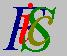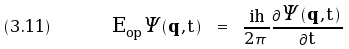Mauro Murzi's pages on Philosophy of Science - Quantum mechanics
Second Schrödinger equation

# [3. Schrödinger equations.]

## Second Schrödinger equation.

The second Schrödinger equation describes the time dependence of Ψ (q):.

where Eop is the quantum operator for the energy.

If Ψ (q) is known at a given time, then Ψ (q) can be calculated at every time in the future and in the past, by means of the second Schrödinger equation. Thus, in the state S, the probability of every physical quantity is determined in every time.